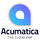# Ideas for Acumatica

## Multi-Level Discounts

Multi Level Discount is a common practice of some of our clients especially for wholesale distributors where multiple discounts are applied to an item/order. Multi-Level Discount is illustrated below.

Suppose I have the following details on purchase order for Item X:
Order Qty: 3
Unit Cost: 60
Ext. Cost: 180
Discount Given: 2%, 3%, 4%

Above discount has 3 levels which is computed as follows:
For 2%:
Level 1 Discount: 180 * 0.02 = 3.6
Level 1 Net Cost: 180 - 3.6 = 176.4

For 3%:
Level 2 Discount: 176.4 * 0.03 = 5.292
Level 2 Net Cost: 176.4 - 5.292 = 171.108

For 4%:
Level 3 Discount: 171.108 * 0.04 = 6.84432
Level 3 Net Cost: 171.108 - 6.84432 = 164.26

Therefore the Order Total is 164.26
As can be seen from above illustration, the net cost of each level depends on the result of net cost from the previous level.

Note: Multi-Level Discounts can also be applied in a Sales Order for customer.
•Gherley Joy Lois Cello
• Nov 9 2017
•